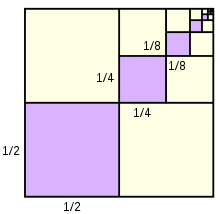# GP sequence

Find the remaining unknown characteristics in the finite geometric sequence, if given: a1 = 5, an = 320, sn = 635, n =?, q =?

n =  7
q =  2

### Step-by-step explanation:Did you find an error or inaccuracy? Feel free to write us. Thank you!

Tips to related online calculators
Do you have a linear equation or system of equations and looking for its solution? Or do you have a quadratic equation?